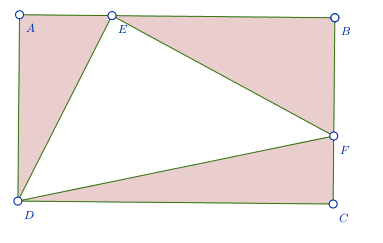# Areas Inside a Rectangle

Geometry Level 4$ABCD$ is a rectangle with point $E$ on line segment $AB$ and point $F$ on line segment $BC$ such that $[ADE] = 7$, $[BEF] = 11$ and $[DCF] = 9$. Then $[DEF] = a\sqrt{b}$, where $a$ and $b$ are integers and $b$ is not a multiple of the square of any prime. What is the value of $a + b$?

Details and assumptions

$[PQRS]$ denotes the area of figure $PQRS$.

×